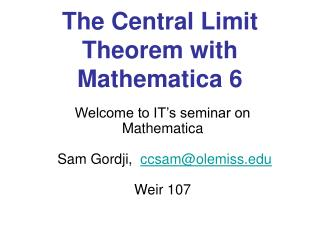DownloadDownload PresentationThe Central Limit Theorem with Mathematica 6

# The Central Limit Theorem with Mathematica 6

Download Presentation## The Central Limit Theorem with Mathematica 6

- - - - - - - - - - - - - - - - - - - - - - - - - - - E N D - - - - - - - - - - - - - - - - - - - - - - - - - - -
##### Presentation Transcript

1. The Central Limit Theorem with Mathematica 6 Welcome to IT’s seminar on Mathematica Sam Gordji, ccsam@olemiss.edu Weir 107

2. General Information • Questions will be answered during the seminar • What is Mathematica: • Mathematica does mathematical operations • Its competitors are MathWorks, Maple, and others • University decided on getting Mathematica after looking into other similar packages